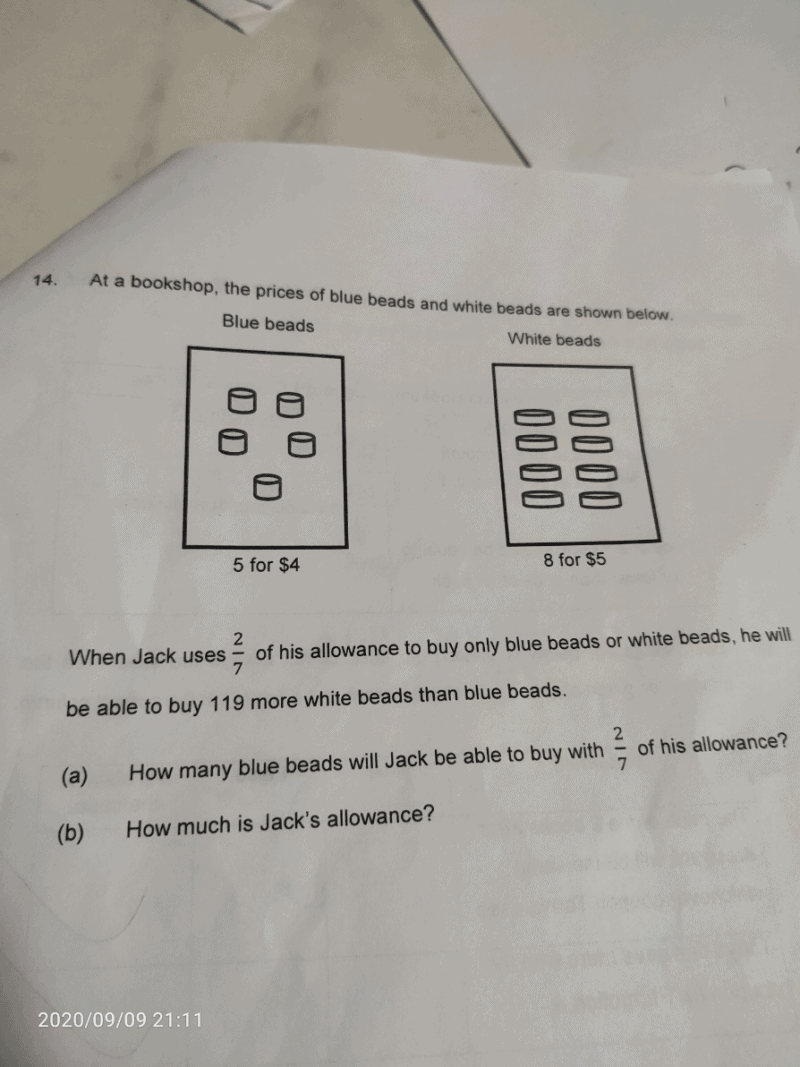# QuestionAnyone pleas help with this question,🙏🙏🙏

Number of blue beads → u

Number of sets of blue beads → u ÷ 5 = u/5

Cost of blue beads →  u/5 × 4 = 4u/5

Number of white beads → u + 119

Number of sets of white beads → (u + 119) ÷ 8 = (u + 119)/8

Cost of white beads →  (u + 119)/8 × 5 = (5u + 595)/8

4u/5 = (5u + 595)/8

32u = 25u + 2975

7u = 2975

u = 425

(a) Jack could buy 425 blue beads with 2/7 of his allowance

(b)

Cost of blue beads → 4u/5 = 4 × 425 ÷ 5 = \$340 (2/7 of his allowance)

2/7 = \$340

7/7 = \$340 ÷ 2 × 7 = \$1190